# MCAT Physical : Voltaic/Galvanic Cells

## Example Questions

### Example Question #15 : Electrochemistry

A student conducts an experiment for a chemistry class. The student wishes to explore power generation from different types of voltaic cells. He sets up three different cells, and then compares the amount of energy generated from each one.

One of his cells is shown below as an example. Both remaining cells follow the same layout.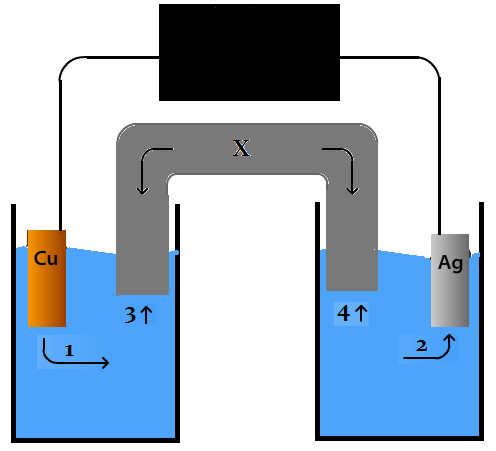In the above diagram, a KOH salt bridge is used. Which species would you anticipate finding at the tip of the arrow numbered 4?

The reduction potential for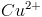is 0.34. The reduction potential for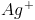0.80.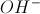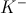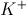Explanation:

The correct answer is, as we would need to balance the loss of positive silver ions in that half cell. Silver has the greater reduction potential, and is therefore gaining electrons to become more negative. As the negative charge develops, it will attract the positive potassium ions in the salt bridge.

### Example Question #16 : Electrochemistry

A student conducts an experiment for a chemistry class. The student wishes to explore power generation from different types of voltaic cells. He sets up three different cells, and then compares the amount of energy generated from each one.

One of his cells is shown below as an example. Both remaining cells follow the same layout.The student in the passage creates a new cell, but uses the same element at both the anode and the cathode. What is likely to be true of this new cell?

Reduction and oxidation are not occuring in the cell

The Gibbs free energy of this cell is zero

This cell can generate energy, but only after energy is input into the system

This cell cannot generate energy

Different concentrations of the species are present in each cell

Different concentrations of the species are present in each cell

Explanation:

This is an example of a concentration cell. If you have two half cells, each made of the same chemical species, and connect them with a wire, the cell will generate a voltage as it attempts to correct the disequilibrium induced by the concentration difference.

### Example Question #17 : Electrochemistry

A student conducts an experiment for a chemistry class. The student wishes to explore power generation from different types of voltaic cells. He sets up three different cells, and then compares the amount of energy generated from each one.

One of his cells is shown below as an example. Both remaining cells follow the same layout.The student in the passage wants to replace the water, represented by the blue in the diagram, with an alternative solvent. Which solvent would be most likely to produce a functioning cell?

Heptane

Benzene

Carbon tetrachloride

Ethane

Acetic acid

Acetic acid

Explanation:

The function of a voltaic cell requires the generation and dissolution of ions. Acetic acid is the only answer choice with a net dipole moment, and would therefore be the only one to dissolve the ions produced. The other choices would be unable to dissolve the ions, and the cell would not function.

### Example Question #18 : Electrochemistry

A student conducts an experiment for a chemistry class. The student wishes to explore power generation from different types of voltaic cells. He sets up three different cells, and then compares the amount of energy generated from each one.

One of his cells is shown below as an example. Both remaining cells follow the same layout.If the structure labeled X were removed from the cell, which of the folllowing would be true?

The reduction potential foris 0.34. The reduction potential for0.80.

Copper ions forming during cell function would be increasingly repelled by existing ions in solution

Copper ions forming during cell function would be increasingly attracted by existing ions in solution

Ag ions forming during cell function would be inceasingly attracted by existing ions in solution

Ag ions forming during cell function would be inceasingly repelled by existing ions in solution

The cell would generate an uncontrolled burst of high energy

Copper ions forming during cell function would be increasingly repelled by existing ions in solution

Explanation:

As the cell runs, only copper produces ions. Silver actually loses ions from solution. If this continues without balance by the salt bridge, the positive ions build up in the solution on the left side of the diagram, without any balanced negative ions, and repel the formation of new copper ions more and more strongly.

### Example Question #19 : Electrochemistry

A student conducts an experiment for a chemistry class. The student wishes to explore power generation from different types of voltaic cells. He sets up three different cells, and then compares the amount of energy generated from each one.

One of his cells is shown below as an example. Both remaining cells follow the same layout.The student in the passage disassembles the cell, and attempts to rebuild it the next day. He forgets, however, to includein the solvent of the half cell on the right side of the diagram. Which of the following is likely to happen?

The cell will produce more energy, because there is no existing ion to repel further ionization into solution

The cell requires, not, in the right-side half reaction to function properly

The cell will not function at all

The cell will function, but for a shorter period of time before it reaches equilibrium and ceases to produce energy

The cell will function withoutpre-existing in solution, but not withoutThe cell will not function at all

Explanation:

There must bepre-existing in the right-side half reaction for the cell to function at all. Without the pre-existing ions, the cell has no electron acceptor dissolved in solution, and no electron transfer can therefore take place. Electron transfer is the fundamental energy-producing process that takes place in a voltaic cell.

### Example Question #20 : Electrochemistry

A student conducts an experiment for a chemistry class. The student wishes to explore power generation from different types of voltaic cells. He sets up three different cells, and then compares the amount of energy generated from each one.

One of his cells is shown below as an example. Both remaining cells follow the same layout.Which of the following compounds could be used to construct the structure labeled "X"?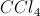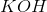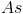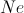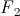Explanation:

Structure "X" corresponds to the slat bridge. Its main function is balancing the ionic charge that builds up as a voltaic cell functions; therefore, there needs to be an ability for the salt bridge to ionize and send appropriate ions to each half cell. KOH is the only ionizable salt among the answer choices.

### Example Question #1 : Voltaic/Galvanic Cells

When a galvanic cell contains two different solutions, a salt bridge is often required. The salt bridge allows the two solutions to remain isolated by providing ionic interactions for both solutions, without severely reducing the potential difference. The salt bridge is commonly composed of a potassium chloride solution.

In what direction would you expect the potassium ions to travel in a galvanic cell?

Toward the anode

The potassium will remain bound to chlorine, and will not ionize

The ions will distribute evenly in the salt bridge

Toward the cathode